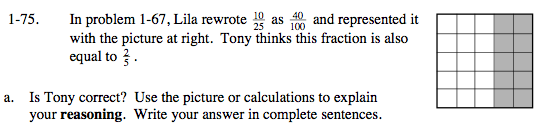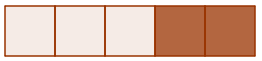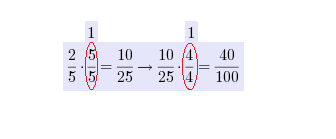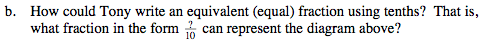### Home > MC2 > Chapter 1 > Lesson 1.2.3 > Problem1-75

1-75.Examine the picture and notice how the square is broken up into 25 square units. If you created a 100 square unit box and shaded in 40 of the squares, would that be the same as having four of the above shapes placed together to form a box?

What about a rectangle with 5 square units and 2 shaded squares? Can the shape above be rearranged to form 5 rectangles like the one pictured below?Each of the farctions are equivalent to each other
because of the multiplicative identity as noted on$\frac{2}{5}\rightarrow \frac{?}{10}$
$\frac{4}{10}$